# Price lists

Price list can be linked on the Dimension option and enable the setup of dynamic prices based on the dimension inputs without the need to implement a price formula.

## Enable extension

Price lists are included in the Price formula Extension.

## Setup

Price lists are defined in the Assets > Lists tab: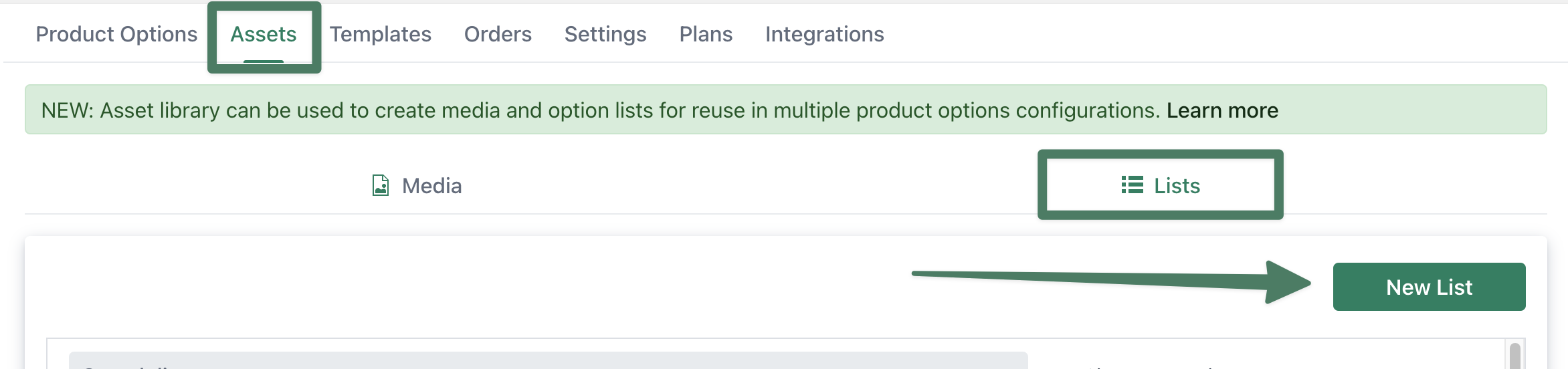### Dimension

Price lists can be linked to the Dimension option, on the Advanced tab: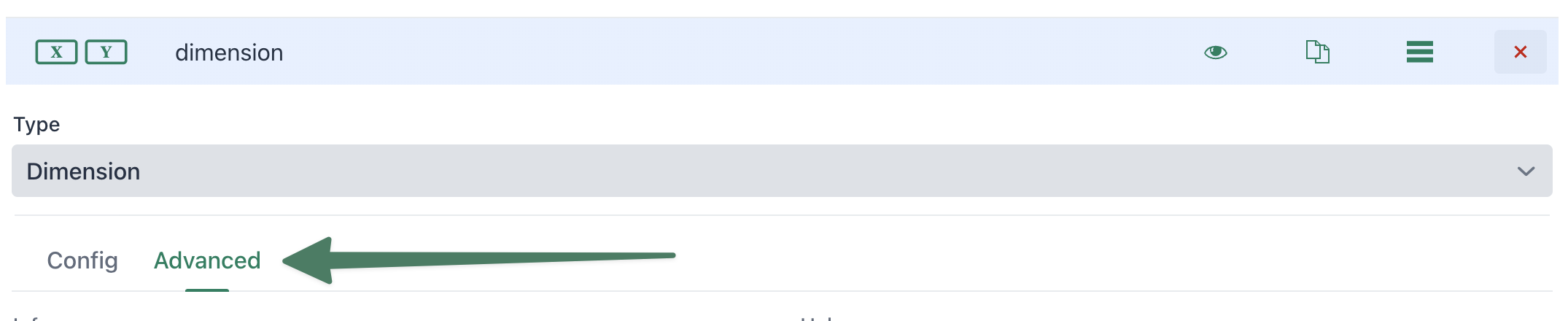There you will find the Price list setting, click on "Link list" to select a previously created Price list: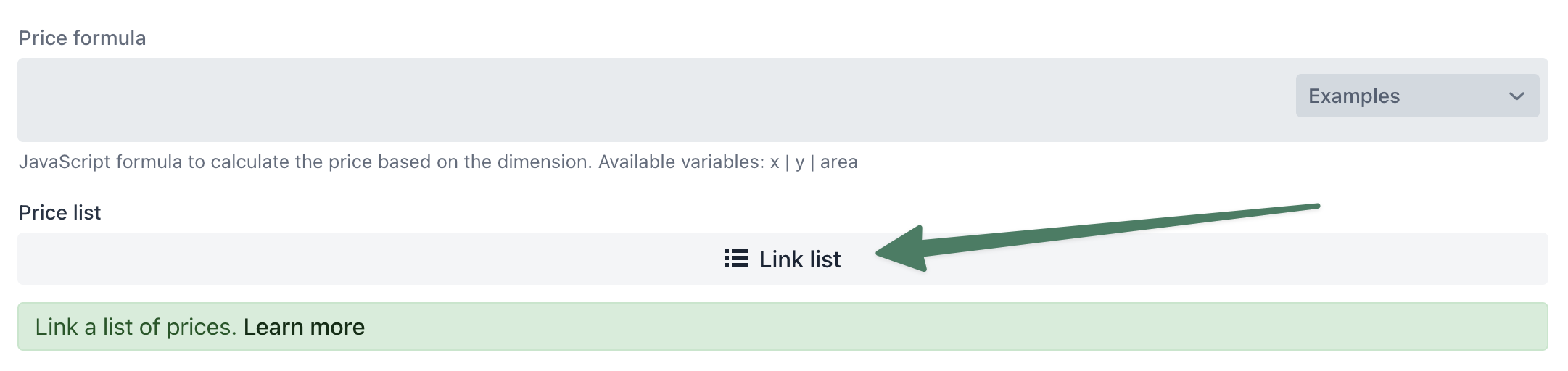IMPORTANT: for the price list to be applied automatically, the Price formula field has to be empty.

If there is a price formula present it will always be preferred over the linked list.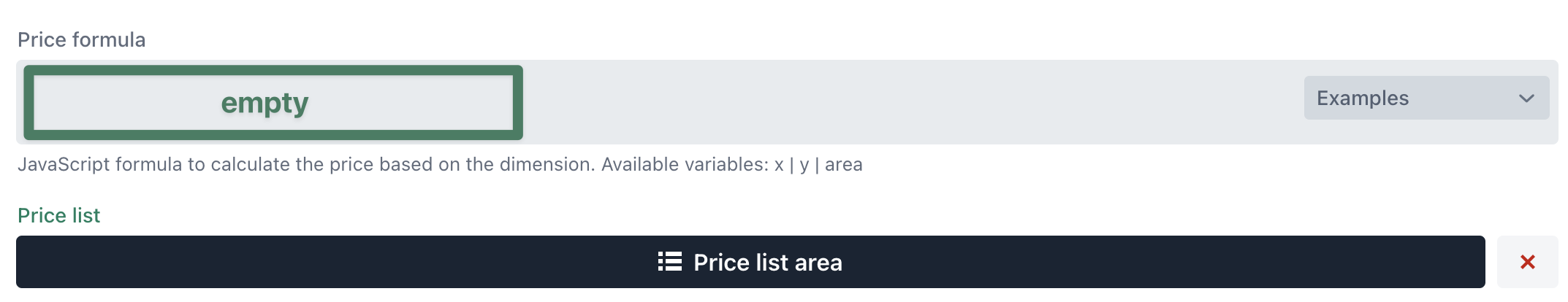Note: You can also combine a price formula with a price list. You can find examples at the end in the section Price formula.

The following sections go into detail on the different type of price lists.

## Price list area

Prices are configured by area. The app finds the matching area range by checking area >=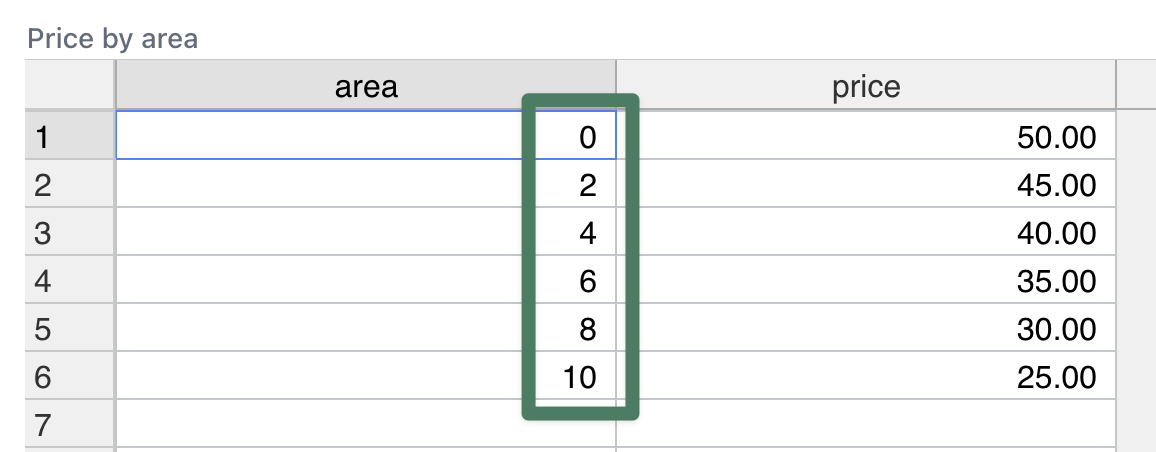The area calculation can be configured on the Dimension option Advanced tab: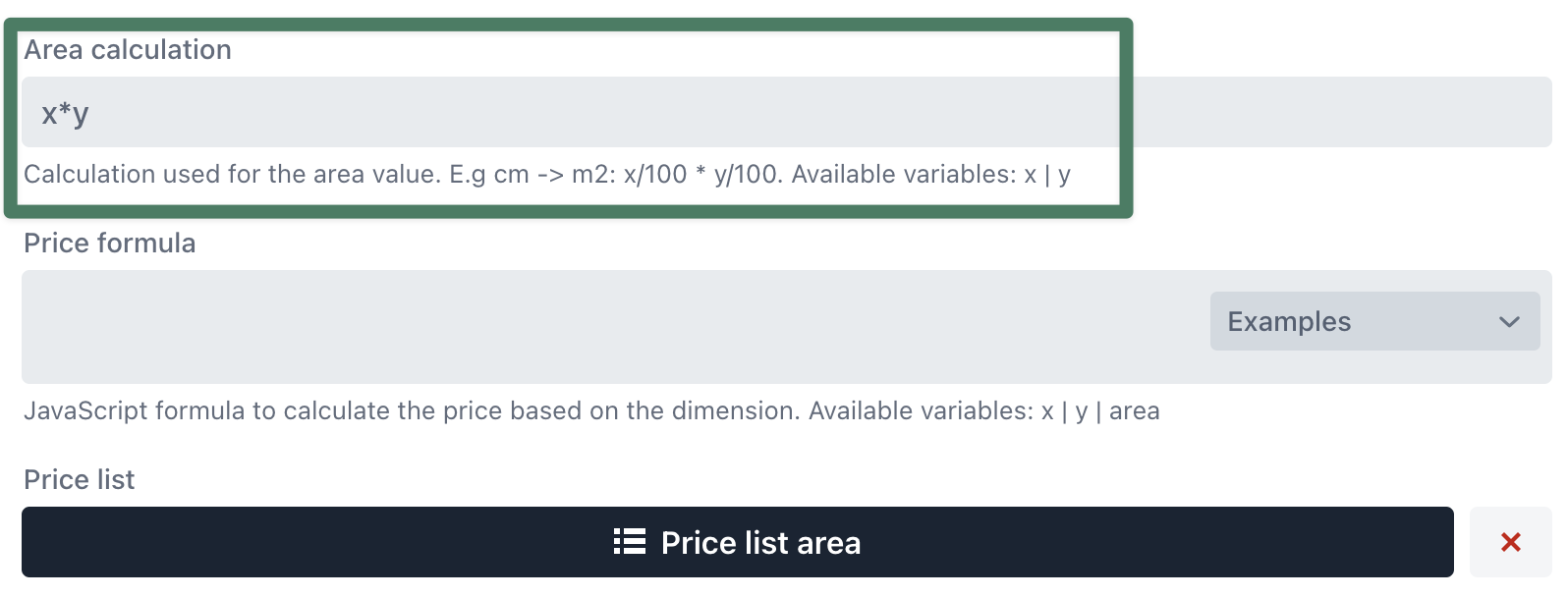## Price list dimension

Prices are configured by X and Y input ranges. The app finds the matching X and Y range by checking >= from the biggest range downwards.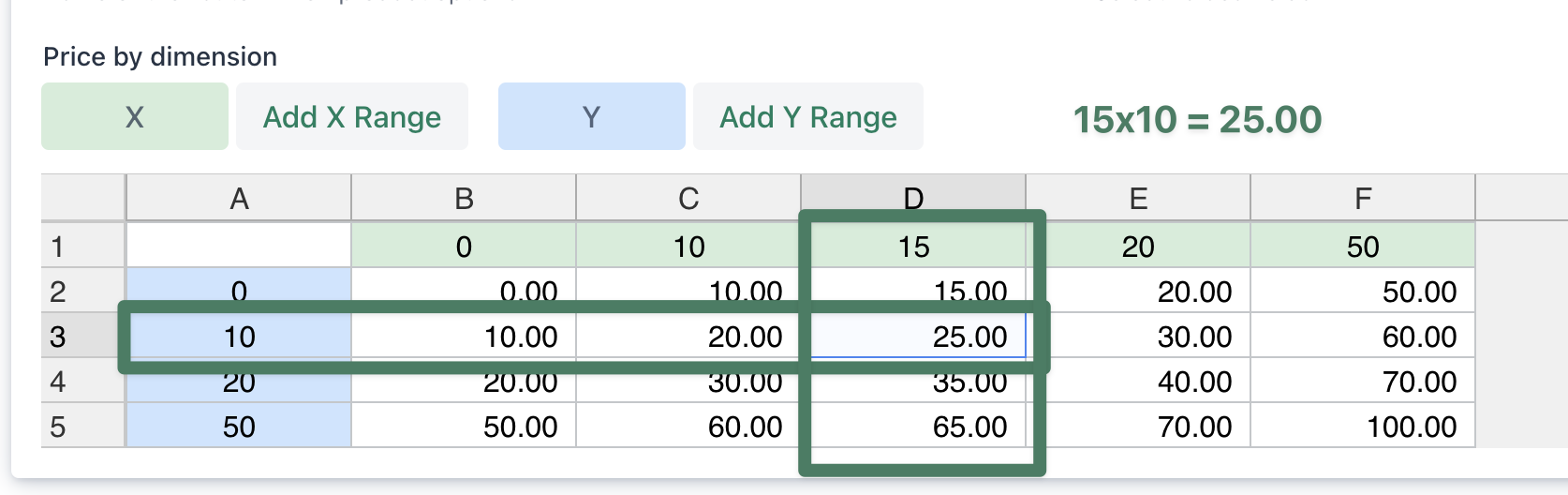## Price formula

For more advanced use cases, you can also lookup a price from the linked price list inside of a Price formula.

The following functions are available inside of a price formula:

Function Description
lookup(area) lookup price by area
lookup(area, option) lookup price by area and option column in price list
lookup(x,y) lookup price by dimension X/Y input

### Area lookup with custom price increase

`// lookup price by area from linked price listvar squarePrice = lookup(area);console.log('square price', squarePrice);// calculate price for the used areavar price = squarePrice * area;// custom price increaseif(x > 80 && y>80){  price += 10;  console.log('adding price increase:', price);}return price;`

Note: the returned price from the lookup function always has to be multiplied by the * area

### Area lookup with option for Material

The Price list area can have one more column that can be used to match for a combination of other selected options. In this example we've setup two different Materials with each their own price per area.

On the price list definition, right click on the header row, and select the "option" column: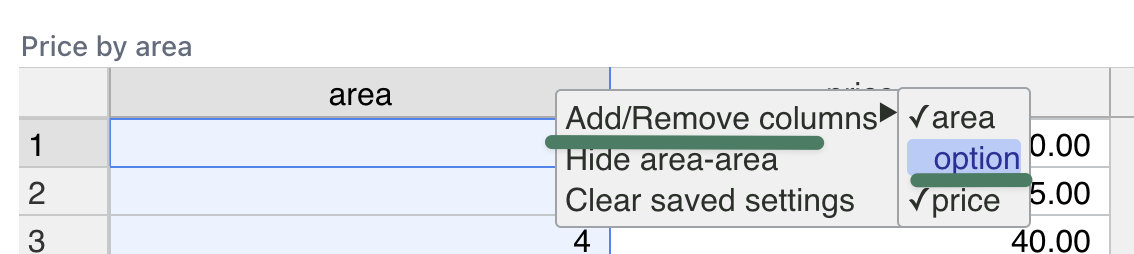Create a row for each area & option combination: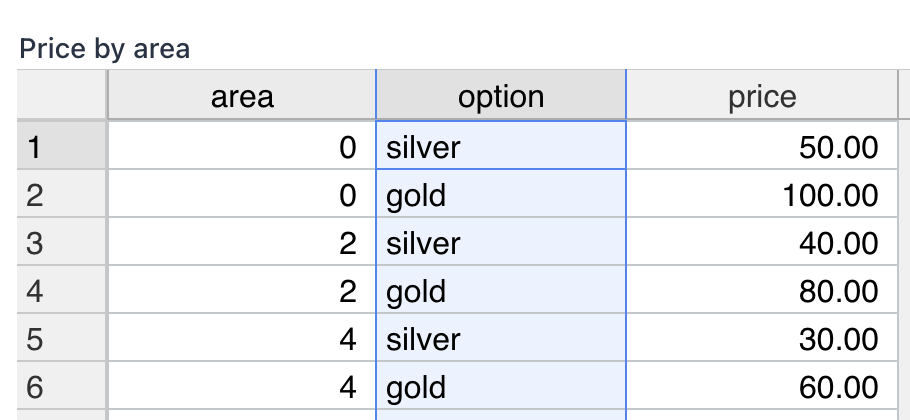In the price formula we first get the selected material, then provide it as a parameter in the lookup function:

`// get the selected material selectionvar material = options.value('material');console.log('square material', material);// lookup price by area & optionvar squarePrice = lookup(area, material);console.log('square price', squarePrice);// calculate price for the used areareturn squarePrice * area;`

Note: you can also combine multiple options into single variable and pass it to the lookup function.